# What is a Derivation tree in TOC?

Derivation tree is a graphical representation for the derivation of the given production rules of the context free grammar (CFG).

It is a way to show how the derivation can be done to obtain some string from a given set of production rules. It is also called as the Parse tree.

The Parse tree follows the precedence of operators.

The deepest subtree is traversed first. So, the operator in the parent node has less precedence over the operator in the subtree.

## Properties

The properties of the derivation tree are given below −

• The root node is always a node indicating the start symbols.
• The derivation is read from left to right.
• The leaf node is always the terminal node.
• The interior nodes are always the non-terminal nodes.

## Example

The production rules for the derivation tree are as follows −

E=E+E
E=E*E
E=a|b|c

Here, let the input be a*b+c

## Step 1

The step 1 is given below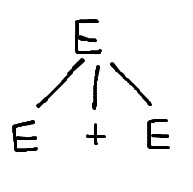## Step 2

The step 2 is given below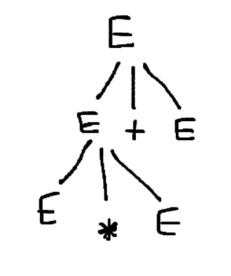## Step 3

The step 3 is given below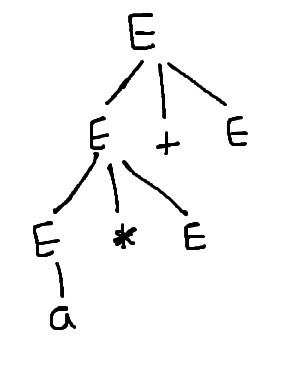## Step 4

The step 4 is given below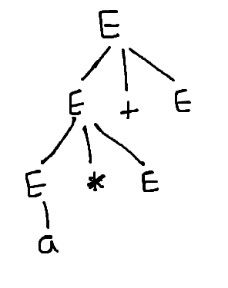## Step

The step 5 is given below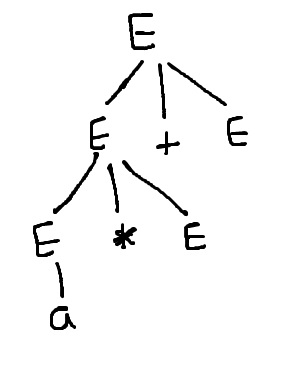Updated on: 11-Jun-2021

11K+ Views